# Verlet algorithm: Why am I getting this output?

• H Smith 94

#### H Smith 94

Gold Member
Hi! I am currently trying to write a code in C to simulate the orbit of planets around the Sun in the solar system.

I am using the velocity Verlet approach and finding that my code produces no acceleration in the ##y##-direction (aside from for ##t_n = 0##,) and that the planet just flies off to infinity without finding a stable orbit.

C:
   /*   INITIALIZATION */

int N = 366;       // Maximum number of steps taken
if(N >= MAX_STEPS)   {   // Error handling
printf("N = %3i is greater than the alloted array space: %4i. \nPlease use smaller N or increase size of arrays.", N, MAX_STEPS);
exit(0);
}

double dt = 1.0 / 365.4;   //   Integration step

/*Initial conditions*/
t0     = 0.0;
t    = t0;
t[-1]    = t0 - dt;

r    = 1.0;
th   = 45*deg;

x    = sqrt(pow(r,2)/(1+pow(tan(th),2)));         // Setting initial position of planet
y    = sqrt(pow(r,2)*pow(tan(th),2)/(1+pow(tan(th),2)));

double v0;
//   v0   = 2.0*M_PI*Au/yr;
v0    = sqrt(G*M/r);
vx0   = v0*cos(th);
vy0   = v0*sin(th);

//   ax = - (G*M/pow(r,2))*cos(th);
//   ay = - (G*M/pow(r,2))*sin(th);

x[-1] = x - vx0*dt;           // Defining x for pre-leap
y[-1] = y - vy0*dt;           // Defining y for pre-leap

printf("\tr(-1) = (%f, %f)\n",
x[-1], y[-1]);

/********************/
/* VERLET ALGORITHM */
/*__________________*/

int n;                   //   Iteration variable
FILE *f = fopen(filename, "w");       //   Opening output file in "write" mode
for(n = 0; n <= N; n++)     {

r[n] = sqrt(pow(x[n],2) + pow(x[n],2));

ax[n] = - G*M*cos(th[n])/pow(r[n],2);
ay[n] = - G*M*sin(th[n])/pow(r[n],2);

x[n+1] = 2*x[n] - x[n-1] + ax[n]*pow(dt,2);         //   Calls function to step x from x[n] -> x[n+1]
y[n+1] = 2*y[n] - y[n-1] + ay[n]*pow(dt,2);

fprintf(f,"%3.4f\t%3.4f\t%3.4f\n",
x[n], y[n], t[n]);         // Prints plot variables (x,v,t) to file
printDiagnostics(x, y, ax, ay, t, n);   // Calls subroutine to print diagnostics to Console

t[n+1] = t[n] + dt;           //   Defines time at iteration n; discrete time Tn = n*dt
}
fclose(f);                 // Closing output file

return 0;                 // To satisfy integer requirement of function
}

This code produces the following output graph: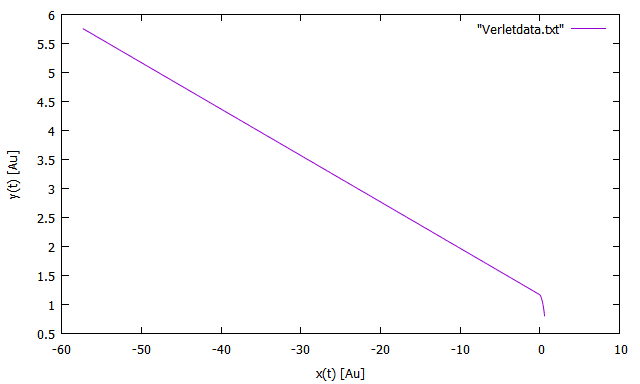Fig. 1
Output from broken simulation showing no stable planetary orbit.
Note that the Sun is placed at (0,0) and we are examining the Earth's orbit.
I've also provided the full output file from the software as an attachment; the first few lines of diagnostics output are as follows:

Code:
         r(-1) = (0.601989, 0.792215)
n = 0 | r(0) = (0.591813, 0.806075)   -> 0.836950    | a(0) = (33.353809, -45.429385)   t(0) = 0.000000
n = 1 | r(1) = (0.581886, 0.819596)   -> 0.822911    | a(1) = (-58.298016, -0.000000)   t(1) = 0.002737
n = 2 | r(2) = (0.571523, 0.833117)   -> 0.808255    | a(2) = (-60.431386, -0.000000)   t(2) = 0.005473
n = 3 | r(3) = (0.560707, 0.846637)   -> 0.792959    | a(3) = (-62.785279, -0.000000)   t(3) = 0.008210
##\vdots##                      ##\vdots##                ##\vdots##                    ##\vdots##                ##\vdots##              ##\vdots##

Is anyone able to see where my code is going wrong? Clearly these graphs do not show a stable orbit!

I am using the MinGW GCC compiler through Eclipse Mars on Windows 8.

It's probably worth noting that this is a homework question, so I'm looking mainly for hints and advice. Thanks in advance!

Last edited:

Have you tried changing your timestep?
If it's too large you'll lose a lot of accuracy.

If I recall correctly I used around 90000 seconds (25 hrs) when I did a similar thing for Halley's comet.
It should be noted however that the period of the comet is much larger, about 75 years.

The second thing I noticed is that your algorithm doesn't look like the velocity verlet algorithm.
When running into problems like this I would always start from the most explicit version of the algorithm.
You can try to simplify it, although I doubt it can get any simpler.

Just out of curiosity - why are you using negative index values like x[-1]? Did you increment x as a pointer and then want to get the value x? It is not illegal syntactically but you can possibly get undefined behavior and/or weird answers if the code doesn't segfault (raise a memory access exception).

Example:
Code:
 x[-1] = x - vx0*dt;           // Defining x for pre-leap
y[-1] = y - vy0*dt;           // Defining y for pre-leap

The immediate error is here:
C:
     ax[n]=- G*M*cos(th[n])/pow(r[n],2);
ay[n]=- G*M*sin(th[n])/pow(r[n],2);

You are never setting th[n]. Given the behavior, the array is filled with zeroes. Since ##\sin(0)=0##, you are getting no acceleration in the y direction. You should either compute each th[n], or better yet, use a different algorithm. You should instead be using ##\vec a(t) = -\frac {\mu}{||\vec r(t)||^3} \vec r(t)## calculate your accelerations, where ##\mu## is conceptually the equivalent of ##GM##. In code,
C:
     rsq = x[n]*x[n] + y[n]*y[n];
r[n] = sqrt(rsq);
fact = -mu/rsq;
ax[n] = fact*x[n];
ay[n] = fact*y[n];
x[n+1] = 2*x[n] - x[n-1] + ax[n]*dtsq;
y[n+1] = 2*y[n] - y[n-1] + ay[n]*dtsq;

I'm using some auxiliary variable in the above. rsq is the square of the radius, ##||\vec r(t)||^2 = x^2 + y^2##, fact is ##-\frac{\mu}{||\vec r(t)||^3}##, mu is ##\mu## (see above and below), and dtsq is the square of the time step, calculated once outside the loop.

Note also that I don't call pow. That's an old habit. In C and C++, it's better to use x*x instead of pow(x,2).

Regarding the use of mu. The gravitational constant ##G## is only known to four places of accuracy. The standard gravitational parameter of the Sun is known to more than ten places.

Last edited by a moderator:
•H Smith 94, Silicon Waffle and jim mcnamara

Have you tried changing your timestep?
If it's too large you'll lose a lot of accuracy.

If I recall correctly I used around 90000 seconds (25 hrs) when I did a similar thing for Halley's comet.
It should be noted however that the period of the comet is much larger, about 75 years.

I was aware is had to be small but I didn't know it was that small! I've decreased it to about 1/10th of a day and it has indeed improved the accuracy. I am still getting similarly strange output, as follows.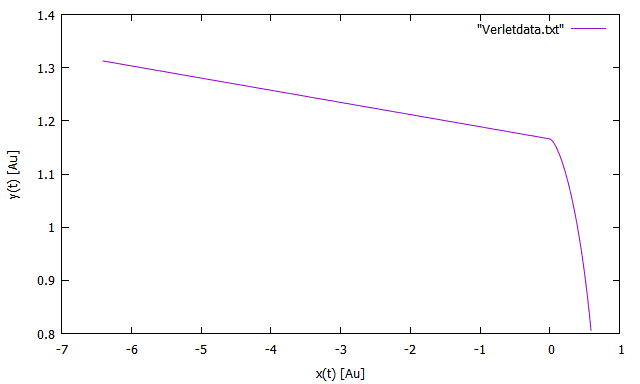Fig. 2
Output with timestep ##dt = 1/3650##.​

The second thing I noticed is that your algorithm doesn't look like the velocity verlet algorithm.
When running into problems like this I would always start from the most explicit version of the algorithm.
You can try to simplify it, although I doubt it can get any simpler.

Honestly, I'm quite a newbie at both C programming and computational physics. I attempted to implement the second algorithm listed on that page: $$\mathbf{r}(t+\Delta t) = \mathbf{r}(t) + \mathbf{v}(t) \Delta t + \frac{1}{2} \mathbf{a} \Delta t$$ $$\mathbf{a}(x+\Delta t) = \frac{1}{m} \mathbf{F}(t+ \Delta t)$$ where ##\mathbf{F}(t+ \Delta t)## is Newton's law of gravitation; and $$\mathbf{v} (t+\Delta t) = \mathbf{v} (t) + \frac{1}{2}(\mathbf{a} (t) + \mathbf{a} (t + \Delta t)\,\Delta t.$$
I interpreted this as defining the timestep of the next iteration as ##t_n+1 = t_n + \Delta t## and then replacing ## t + \Delta t ## with the relevant array element ## n+1 ##. Clearly, this hasn't worked -- what would be a better method to 'translate' these equations into a code?

UPDATE:

I have just realized I was in fact not using the above algorithm -- thank you for pointing this out! In this particular code I was using the Verlet equation from my lecture notes (pg. 11). This also answers the following question.

Sorry, I have re-written this code using a million different methods and they're all starting to blur together at this point!

————————————————————————

Just out of curiosity - why are you using negative index values like x[-1]? Did you increment x as a pointer and then want to get the value x? It is not illegal syntactically but you can possibly get undefined behavior and/or weird answers if the code doesn't segfault (raise a memory access exception).

Last edited:
Wow, thank you for your extensive post!

The immediate error is here:
C:
     ax[n]=- G*M*cos(th[n])/pow(r[n],2);
ay[n]=- G*M*sin(th[n])/pow(r[n],2);

You are never setting th[n]. Given the behavior, the array is filled with zeroes. Since ##\sin(0)=0##, you are getting no acceleration in the y direction. You should either compute each th[n], or better yet, use a different algorithm.

Well spotted! I'm a little embarrased I didn't notice that myself. I have now defined th[n] as th[n+1] = atan( y[n+1] / x[n+1]), or $$\theta(t+\Delta t) = \mathrm{arctan}\left(\frac{y(t+\Delta t)}{x(t+\Delta t)}\right).$$
UPDATE:
The following step makes this line of code not-needed. No need to use that pesky atan() function.

You should instead be using ##\vec a(t) = -\frac {\mu}{||\vec r(t)||^3} \vec r(t)## calculate your accelerations, where ##\mu## is conceptually the equivalent of ##GM##. In code,
C:
     rsq = x[n]*x[n] + y[n]*y[n];
r[n] = sqrt(rsq);
fact = -mu/rsq;
ax[n] = fact*x[n];
ay[n] = fact*y[n];
x[n+1] = 2*x[n] - x[n-1] + ax[n]*dtsq;
y[n+1] = 2*y[n] - y[n-1] + ay[n]*dtsq;

I'm using some auxiliary variable in the above. rsq is the square of the radius, ##||\vec r(t)||^2 = x^2 + y^2##, fact is ##-\frac{\mu}{||\vec r(t)||^3}##, mu is ##\mu## (see above and below), and dtsq is the square of the time step, calculated once outside the loop.
Should I instead be using ax[n] = fact*x[n]/r[n] here to account for the vector components?

Note also that I don't call pow. That's an old habit. In C and C++, it's better to use x*x instead of pow(x,2).
I was not aware of this, excellent point! I had figured I should be a little wary of C's built-in functions!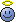Regarding the use of mu. The gravitational constant ##G## is only known to four places of accuracy. The standard gravitational parameter of the Sun is known to more than ten places.
Thank you, these are all great pointers! I have updated my code to reflect this and will keep this in mind in future.

My code now outputs the following, which I assume will start working with the correct selection of initial conditions?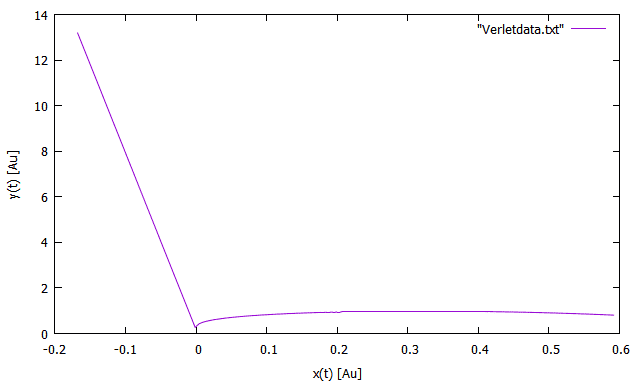Fig. 3
Output with updating th[n].​

Last edited:
I've added my loop from the MATLAB code I have laying around. (it's almost the same as C, I will assume you get the gist of it)
You'll notice I never used the goniometric functions meaning I circumvented the issue D H noticed with your code.

First let me clarify the velocity-verlet algorithm (VVM).

A naive way to integrate the equations of motion (which is what we are actually doing) is the one you are using.
It's called the Störmer-Verlet algorithm.
Basically you give some initial values and then start iterating using the rule
##x_{n+1}=2x_n- x_{n-1}+ a^{(x)}_n\Delta t##
Here I defined ##a^{(x)}## as the x-component of the acceleration.
The velocity has been effectively eliminated from this method.

The VVM however uses the velocity in a very explicit way.
The algorithm consists of some extra steps.

1. Calculate ##v^{(x)}(t+\frac{1}{2}\Delta t) = v^{(x)}(t) + a^{(x)}(t) \frac{1}{2}\Delta t##
2. Calculate ##x(t+\Delta) = x(t) + v^{(x)}(t+\frac{1}{2}\Delta t)##
3. Find ##a(t+\Delta t)## using the interaction potential, in this case the gravitational potential
4. Update ##v(t+\Delta t) = v^{(x)}(t+\frac{1}{2}\Delta t) + a^{(x)}(t+\Delta t) \frac{1}{2}\Delta t##
Notice how I neglected to save the first step and plugged it directly into the second step.
This isn't a very good idea, since I calculate the same thing twice each iteration.
For this simple example this doesn't give us much if any trouble. For larger simulations you want to avoid that.

Matlab:
for ii = 2:N

r(ii,1) = r(ii-1,1)+v(ii-1,1)*Dt+Ax/2*Dt^2;
r(ii,2) = r(ii-1,2)+v(ii-1,2)*Dt+Ay/2*Dt^2;
R = sqrt(r(ii,1)^2+r(ii,2)^2);

%First velocity update
v(ii,1) = v(ii-1,1)+Ax/2*Dt;
v(ii,2) = v(ii-1,2)+Ay/2*Dt;

%Update acceleration
Ax = (-G*M/R^2)*(r(ii,1)/R);
Ay = (-G*M/R^2)*(r(ii,2)/R);

%Second velocity update
v(ii,1) = v(ii,1)+Ax/2*Dt;
v(ii,2) = v(ii,2)+Ay/2*Dt;

%Energy
K(ii) = 1/2*m*(v(ii,1)^2+v(ii,2)^2);
V(ii) = -G*m*M/R;
end

EDIT: (crosspost)
Your latest figure seems to point towards a division by a very small number.

•H Smith 94
I've added my loop from the MATLAB code I have laying around. (it's almost the same as C, I will assume you get the gist of it)
This is a very useful resource! I'm a little wary about implementing half-steps at this point but it has certainly helped me relate the code to the math, thank you.

Your latest figure seems to point towards a division by a very small number.
I thought the same thing, too! But I can't seem to find where it would be, unless 1.0 Au counts as a small number for the initial position r. If you can see anything blaringly obvious, my updated code is as follows:
C:
  /********************/
/* VERLET ALGORITHM */
/*__________________*/

int n;                   //   Iteration variable
FILE *f = fopen(filename, "w");       //   Opening output file in "write" mode
for(n = 0; n <= N; n++)     {

r[n] = sqrt(pow(x[n],2) + pow(y[n],2));

double dtsq = dt*dt;
double rsq  = r[n]*r[n];
double xhat = x[n]/r[n];
double yhat = y[n]/r[n];

ax[n] = - (G*M/rsq)*xhat;
ay[n] = - (G*M/rsq)*yhat;

x[n+1] = 2*x[n] - x[n-1] + ax[n]*dtsq;         //   Calls function to step x from x[n] -> x[n+1]
y[n+1] = 2*y[n] - y[n-1] + ay[n]*dtsq;

//     th[n+1] = atan( y[n+1] / x[n+1]);

fprintf(f,"%3.4f\t%3.4f\t%3.4f\n",
x[n], y[n], t[n]);         // Prints plot variables (x,v,t) to file
printDiagnostics(x, y, ax, ay, t, n);   // Calls subroutine to print diagnostics to Console

t[n+1] = t[n] + dt;           //   Defines time at iteration n; discrete time Tn = n*dt
}

Last edited:
Thank you for all your help everyone!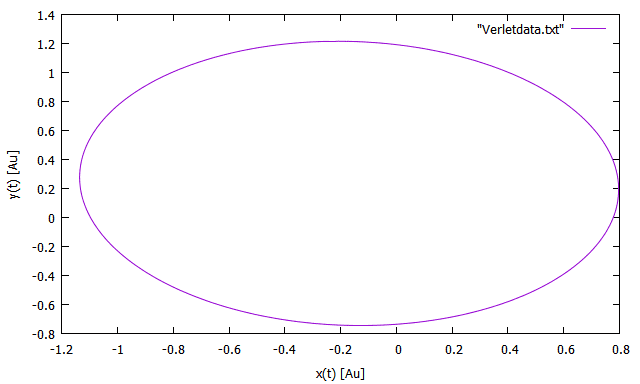Fig. 4
Complete orbit of the Earth around the Sun in 1 year.​

I have attached the full code as a .txt file for anyone interested.

#### Attachments

• VerletSim_C.txt
7.3 KB · Views: 472